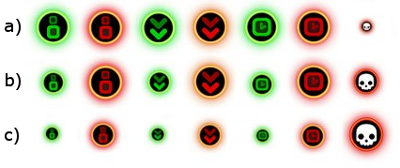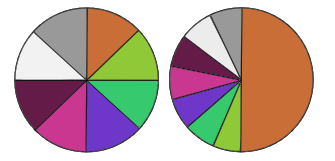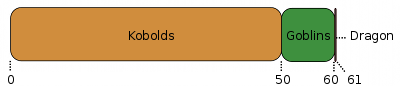## Roulette Wheel Selection Algorithm in MATLAB

I’m not a MATLAB expert myself, but I had to code the roulette wheel selection algorithm, once again, this time in the MATLAB programming language. It complements this post, so I thought I could share it:

```% ---------------------------------------------------------
% Roulette Wheel Selection Algorithm. A set of weights
% represents the probability of selection of each
% individual in a group of choices. It returns the index
% of the chosen individual.
% Usage example:
% fortune_wheel ([1 5 3 15 8 1])
%    most probable result is 4 (weights 15)
% ---------------------------------------------------------
function choice = fortune_wheel(weights)
accumulation = cumsum(weights);
p = rand() * accumulation(end);
chosen_index = -1;
for index = 1 : length(accumulation)
if (accumulation(index) > p)
chosen_index = index;
break;
end
end
choice = chosen_index;```

It can be probably rewritten in a nice MATLAB way, but it’s useful as is.

## Roulette wheel selection for procedural content generation

There are times when you need to give your code the ability to choose among many options that have different frequencies or probabilities of being selected. Whether you need to create a random power-up or decide the next enemy encounter, here’s a tool that you will find useful: the Roulette Wheel selection algorithm, or Fitness Proportionate Selection. While it is a common operator in Genetic Algorithms, it is also very handy for videogame programmers!

First of all, an example

In Sound Juggler, the Roulette Wheel selection algorithm was used to choose which powerup would appear next. There were seven of them: three were ‘good’, three were ‘bad’ and one caused ‘sudden death’. Initially, all of them have the same probability of being chosen except for the sudden death one, which is quite rare (See Fig. 1.a, where probabilities are represented as icon sizes). However, as the game continues, negative powerups (in red) become increasingly more frequent (Fig. 1.b-1.c).  With Roulette Wheel selection, choosing the next powerup is really easy.As the game advances, powerup probabilities change (size is proportional to probability)

Size does matter

Quoting Wikipedia: “The analogy to a roulette wheel can be envisaged by imagining a roulette wheel in which each candidate solution represents a pocket on the wheel; the size of the pockets are proportionate to the probability of selection of the solution.”

On a given wheel all pockets may have equal size and thus equal probability of being chosen: 1 / n, being n the number of pockets (see the left roulette in Fig.2).  Now, imagine that one of the pockets occupies half the space (right roulette in Fig.2). It is easy to see that the roulette will stop inside it many more times than in any other pocket. Statistically, its probability is exactly 0.5, although you don’t really need to know it if you understand how this works.In the left wheel, all pockets have the same size. In the right wheel, the orange pocket is seven times bigger than any other pocket (and thus, seven times more probable!)

So, whenever you have a set of choices with different probabilities, you simply have to assign them the right pocket size and spin the wheel!  Of course, the algorithm does not use a ‘wheel’ per se.

Programming the Roulette

We will use Unity Script, which is similar to Javascript. First of all, we need a roulette, a place to store probabilities. It will be defined by an array of floats.

`var roulette: float[];`

Then we initialize it with each pocket probability. Say we have four items to be chosen. If they are equiprobable, our roulette should look like this:

```roulette = new float;
roulette = 1.0;
roulette = 1.0;
roulette = 1.0;
roulette = 1.0;```

Ok, we call them ‘probabilities’ although they do not sum 1.0. We could simply normalize values dividing each one by the sum of all of them. But we can ignore that part safely.

Now, if we wanted the first item to be two times more probable than any other, we simply have to set values this way:

```roulette = new float;
roulette = 2.0; <-- 2.0 / 5.0 = 0.4
roulette = 1.0; <-- 1.0 / 5.0 = 0.2
roulette = 1.0;
roulette = 1.0;```

Easy! See how it works? Those numbers can be understood as the region that each pocket occupies in the roulette. The first pocket is two times bigger than any other, increasing its probability of being chosen. Now, how do we spin the wheel? First of all, we sum all pocket values:

```var total : float = 0;
var i : int = 0;
for (i = 0; i < roulette.length; i++)
total = total + roulette[i];```

Done. Then, we chose a random number between 0 and total.

`var goal_value: float = Random.Range(0, total);`

Why? Well, here’s the tricky part.  In order to choose a pocket, we traverse the roulette accumulating pocket values. When this accumulated value is greater than our random goal_value, we have our pocket!

```var sum : float = 0;
for (i = 0; i <= roulette.length-1; i++) {
sum += fortune_wheel[i];
if (sum > goal_value)
break;
}```

And that’s it! After that last for loop, i contains the index of the chosen pocket. Then it’s up to you what to do with it. In Sound Juggler, we used it to choose the next powerup (note that you can alter roulette values whenever you want). In our next game, Oddy Smog’s Misadventure, we use it to select which gear will be placed next (however, not all gears are available at the beginning, so we traverse our roulette from zero to the last allowed gear index).

Choosing the right values

That’s the best part of this algorithm. If you want to sit down and use your knowledge of statistics, you can do it. But if you just want those pesky kobolds to appear inside the Huge Caverns of Dispair five times more frequently than goblins, and the black dragon to be seen around once in a month, you can simply play with numbers until you feel comfortable with results. Graphically, it would look like Fig.3.Dwellers of the Huge Cavern of Dispair. Kobolds are everywhere!

Our code would look like this:

```roulette = new float;
roulette[KOBOLDS] = 50;
roulette[GOBLINS] = 10;
roulette[BLACKDRAGON] = 1;```

In this case, total would sum 61. Most of the time, a random number between 0 and 61 will fall between 0 and 50, and thus a new Kobold will be spawned. But, from time to time, a Dragon will appear to spice up the game. And feast on your corpse.

Use with caution! 🙂